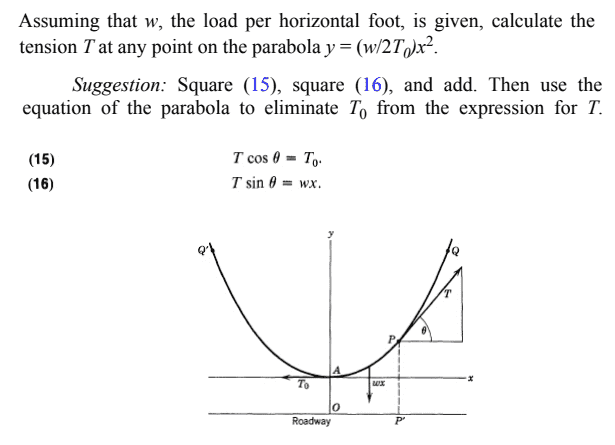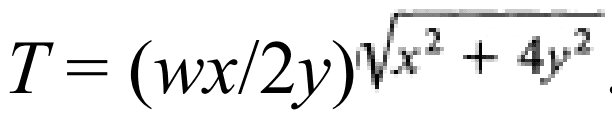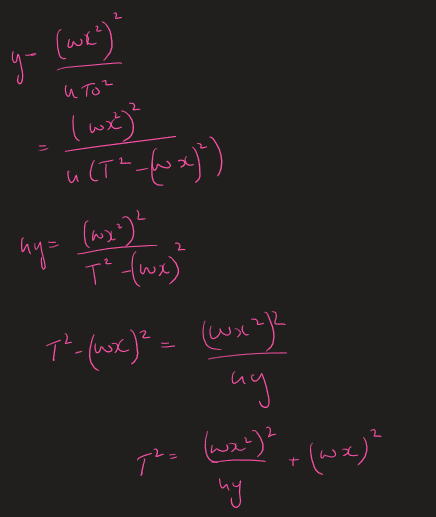# Tension T in a parabolic wire at any point

ElectronicTeaCup
Homework Statement:
Assuming that w, the load per horizontal foot, it given, calculate the tension T
Relevant Equations:
See (15,16) belowI am unsure how to go about this. I tried following the suggestion blindly and end up with with some cumbersome terms that are not the answer. From what I understand the derivative at each point would equal to T?I just can seem to get to this. I think I'm there but can't get it in this formHomework Helper
Gold Member
2022 Award
Homework Statement:: Assuming that w, the load per horizontal foot, it given, calculate the tension T
Relevant Equations:: See (15,16) below

View attachment 262705

I am unsure how to go about this. I tried following the suggestion blindly and end up with with some cumbersome terms that are not the answer. From what I understand the derivative at each point would equal to T?

Answer: View attachment 262706 I just can seem to get to this. I think I'm there but can't get it in this form

View attachment 262707
Right at the start you have y on the left instead of y2. Correct that and you should get the target answer.

•ElectronicTeaCup
What's wrong with their suggestion? I assume you got ##T^2 = T_0^2 + w^2 x^2##, and along with ##y =\frac{w}{2T_0} x^2##. That should be sufficient to find ##T## in terms of ##x##, ##y## and ##w##.

Last edited by a moderator:
•ElectronicTeaCup and Delta2
ElectronicTeaCup
Right at the start you have y on the left instead of y2. Correct that and you should get the target answer.

Yikes! YES, thanks for the catch! I was so frustrated trying to solve this one.